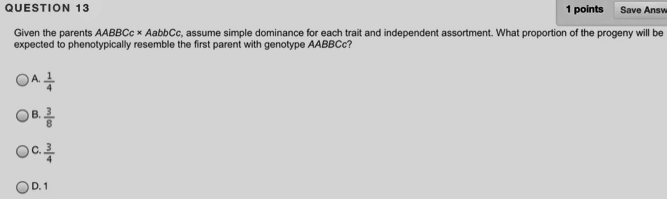# Problem: Given the parents AABBCc x AabbCc, assume simple dominance for each trait and independent assortment. What proportion of the progeny will be expected to phenotypically resemble the first parent with genotype AABBCc?A. 1/4B. 3/8C. 3/4D. 1

🤓 Based on our data, we think this question is relevant for Professor Partridge's class at TEXAS.

###### FREE Expert Solution

In heredity, for a progeny to have a similar phenotype as that of genotype AABBCc, the individual should have the general genotype A_B_C_, where at least one allele for each gene carries the dominant character.

In determining the probability, we can simply multiply the individual probabilities of the desired outcome for each gene. In A/a, the cross was between AA and Aa. This will definitely produce A_ individuals, either AA or Aa. In B/b, the cross was between BB and bb. All offspring would have the genotype Bb, which is phenotypically similar to BB.###### Problem Details

Given the parents AABBCc x AabbCc, assume simple dominance for each trait and independent assortment. What proportion of the progeny will be expected to phenotypically resemble the first parent with genotype AABBCc?

A. 1/4

B. 3/8

C. 3/4

D. 1